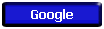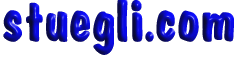# Gravitation and Mechanics

 All objects are attracted toward one another gravitationally The strength of the gravitational force between them depends on their masses and the distance that separates them from one an-other and from the Sun.

### Newton's Principles of MechanicsSir Isaac Newton realized that the force that makes apples fall to the ground is the same force that makes the planets "fall" around the sun.

He established that a force of attraction toward the sun becomes weaker in proportion to the square of the distance from the sun.

Newton postulated that the shape of an orbit should be an ellipse. Circular orbits are merely a special case of an ellipse where the foci are coincident.

Newton's Laws of Motion

1. Every body continues in a state of rest, or of uniform motion in a straight line, unless it is compelled to change that state by forces impressed upon it.
2. The change of motion (linear momentum) is proportional to the force impressed and is made in the direction of the straight line in which that force is impressed.
3. To every action there is always an equal and opposite reaction; or, the mutual actions of two bodies upon each other are always equal, and act in opposite directions.

There are three ways to modify the momentum of a body. The mass can be changed, the velocity can be changed (acceleration), or both.

### Acceleration

Force (F) equals change in velocity (acceleration, A) times mass (M):

#### F = MA

Acceleration may be produced by applying a force to a mass (such as a spacecraft). If applied in the same direction as an object's velocity, the object's velocity increases in relation to an unaccelerated observer. If acceleration is produced by applying a force in the opposite direction from the object's original velocity, it will slow down relative to an unaccelerated observer. If the acceleration is produced by a force at some other angle to the velocity, the object will be deflected. These cases are illustrated below.>Copyright © 2005 -  S. B. EglI